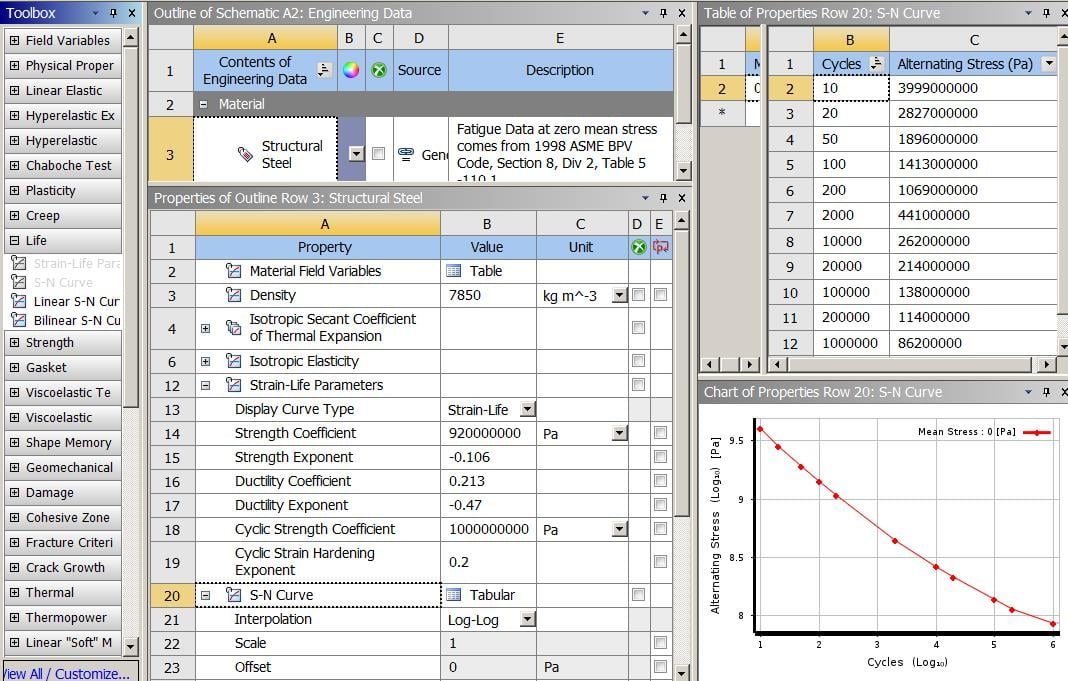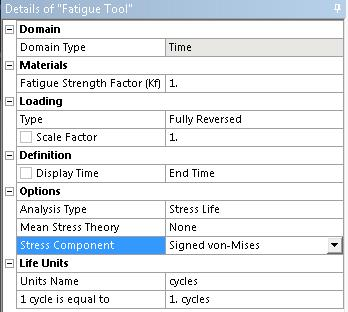## General Mechanical

•rmknox
Subscriber

Hi everyone,

I'm running a fatigue analysis in Static Structural on a number of different orthopedic spine devices. I have four different geometries and am running three tests - compression, torsion, and compression + shear - on each.

The issue I've come across is that the Fatigue Life result is the same for every one of the 12 simulations. In each case, the minimum Life result is reported as 1307300 cycles. I don't doubt this is a realistic result, but I doubt that every single one of the 12 scenarios made it through the exact same number of cycles.

So my question is, how exactly is Life calculated? Is it based off a particular material property? Our device is made of a unique material for which we have reported fatigue data, so the fact that all constructs have the same fatigue result makes me think that the material has something to do with it.

•peteroznewman
Subscriber

Hi rmknox,

As you surmised, ANSYS life data is calculated from values stored for each material.  Look at Structural Steel, which has two types of life data included: Strain-Life and S-N or Stress-Life data.In the simplest terms, life is calculated by taking a Static Structural load which is intended to represent the maximum amplitude of a fully reversed cyclic load and for each value of stress in the model, do a lookup on the S-N Curve and plot the Life. There are many more details to go into, but that is the basic approach.

If you have three load cases, compression, torsion and comp+shear, I expect they each create different maximum values of stress. If all 12 values of maximum stress are less than the lowest stress in the S-N data table, then they will all give the same life. If the stress values are reasonably high, perhaps the S-N data is incorrect.

Regards,
Peter

•rmknox
Subscriber

Hi Peter,

I'm assuming that the maximum stress value you're referring to is the value returned by the Equivalent Alternating Stress tool? If so, they are indeed much less than the lowest stress in the S-N table we plugged in. I'm assuming ANSYS just extrapolates the S-N curve to the relevant stress value? I'm still a little confused as to why the Life values are the same for each test, as the maximum stress values vary by a considerable amount. Is the returned Life value just a placeholder for the "empty space" not covered by the S-N curve, rather than a calculated amount?

Also, the fatigue test we're simulating is an ASTM standard that requires devices be tested out to 5 million cycles. I wasn't sure how/where to plug that number in, but read somewhere that the default fatigue test runs 1 million cycles, so I changed the Life Units so that 1 cycle is equal to 5 cycles. How does this factor in to our result? Is the Life result factoring in this 5:1 expansion? Or is our actual Life result 5 times greater than what the program returned (i.e. the actual Life is 1307300*5 = 6536500 cycles)?

Thank you!

•peteroznewman
Subscriber

Hi rmknox,

The maximum stress I am referring to is whichever stress component you selected in the Fatigue Tool.
There are several to choose from.Don't assume that ANSYS extrapolates the S-N curve to the relevant stress value. It only interpolates. You have to provide a table of stress amplitude and cycles to failure that covers your range of interest. Therefore if you have a "short" table that only goes down to a medium level of stress amplitude and the last value in the table is 1307300, then all analyses with maximum stress levels lower than the last stress amplitude value in the table will report a minimum life as 1307300.

If you have test data out to 5 million cycles, then you type that data in the last row of the S-N table. Do you see the table for steel above goes out to 1 milllion cycles on row 12.  You would enter row 13 as 5 million and the stress amplitude that corresponds to the samples that failed, on average, at 5 million cycles.

If you calculate the six parameters for a Strain-Life equation from your fatigue data and add that to your material, then you will have an equation that can extrapolate out to life values beyond the data, since it's an equation not a table. Read more in the ANSYS Help system.

Life units are going to be 1:1 per cycle unless you are doing some special counting method. Say for example that you were testing a component in a car. The car goes around a test track where one lap is 4 miles long and all the component fatigue data is counted in laps.  Now you want to make a plot that shows the life in miles instead of laps. You can use the factor 4 and now the plot is in miles instead of laps.

Regards,
Peter

•rmknox
Subscriber

Hi Peter,

I see now that the issue is that the material we're using hasn't actually been tested to 5 million cycles - I didn't realize that ANSYS wouldn't extrapolate from S-N data. As you mentioned, our S-N table is "short", so the Life results we got are just the last value in the table. Looks like we need to get some more data from our client.

Thank you very much for your help!